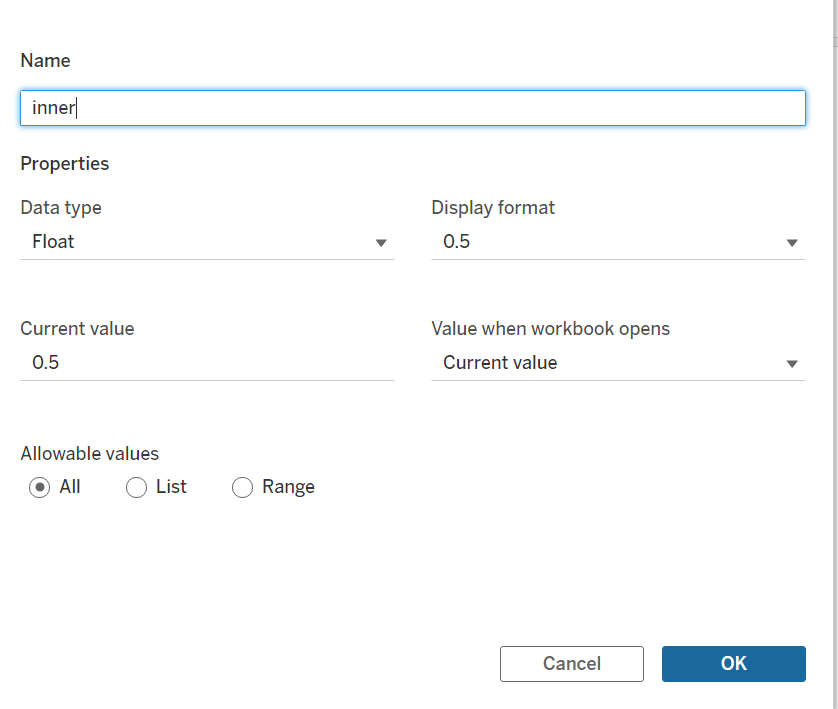top of pageSearch

# HOW TO CREATE A RADIAL LINE CHART IN TABLEAU

The radial line chart is a variation of a pie chart. Like a pie chart, a radial line chart shows the relationship of parts to a whole. Also, a radial line chart can contain sub-categories for each part of the whole. We use a radial line chart when there is a hierarchy in the data like when there is a product category and sub-category.

Basic understanding

Before we start with the steps to create a radial line chart, we need to brush up on our knowledge of trigonometry and geometry. We need to identify a starting and ending point for each of the measure values so that they can be plotted between the points on a circle.

Step 1: Union the data

Let's get the data ready! To create a radial line chart, I am using the Sample -Superstore Data which comes along with Tableau. The original data that needs to be plotted has to be duplicated. Make a union of the table 'Orders' to itself.Create a calculated field,' Path', which holds 1 for one set of the data and 0 for the duplicate data in the sheet.Step2: Create Required Calculations And Parameters

Let us decide on the radii for the inner and outer circle. For this, we can create two parameters, 'inner' and 'outer'.

For the 'inner' radius, we just set the value to 0.5.For the 'outer' radius, we just set the value to 1.After deciding the radii of the 2 circles, we need to decide on the angle at which the measures will be plotted. In a circle, the total angle is 360 degrees or 2 PI radians. So first we assign the index to all the data points from the first to the last row sequentially. Then we divide that by the total count of rows in our table calculation. Then we multiply this by 2 PI so that we will get these values in a full circle.To start with the radial chart, we need 'X' and 'Y' values. Before we use the angle to find the coordinates of the starting and ending points of each line, we need to assign normalized lengths to all the lines.Now create a calculated field for 'X'And 'Y'Step 3: Plot The Chart

Let’s start creating the chart now.

1. Add X to Columns and Y to Rows

2. Choose Line as the mark type on the Marks card

3. Add State to Color on the Marks Card

4. Add the combined field(Category&Month(Order Date)) to Detail on the Marks card

5. Add Path to Path on the Marks Card

6. For X and Y, set the table calculation to Compute Using Order Date

7. Edit the axes so that the range is fixed from -1 to 1

So here the path is a line going from the inner circle( 1st table) to the outer circle(duplicate table). As I keep moving to the right, the angle increases by marginal values which in turn forms a circle. I created the normalized length to make sure the Sum(Sales) for both the tables are on the same scale.

The radial line chart is now ready! Format the chart to remove the Gridlines and zero lines, hide the header, and format the sheet in black color. To make things interesting, I thought to add something in the blank space that we have in the middle. Why not a MAP, right?

Step 4: Plot the MAP

Let's work on a new sheet. Drag State to details and color under marks. Format Map by selecting Map layers from Map . Then select the style to Dark and give black background to match the radial chart.

Step 5: Dashboard

Add the Radial chart and adjust the sizing. Next, add the Map. Change the dashboard formatting to floating so that we can place the Map in the center. Now format, format, and format!!! Once everything goes well, the chart will look like this.

I tried to cover as much as I could for a newbie to get started with such advanced charts. Hope you like it. As always, I welcome feedback and constructive criticism.